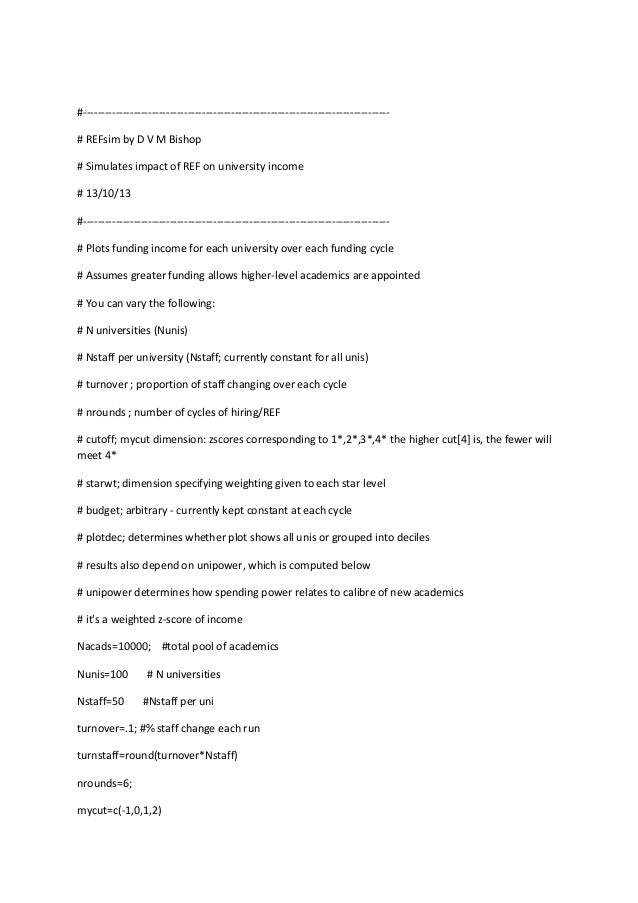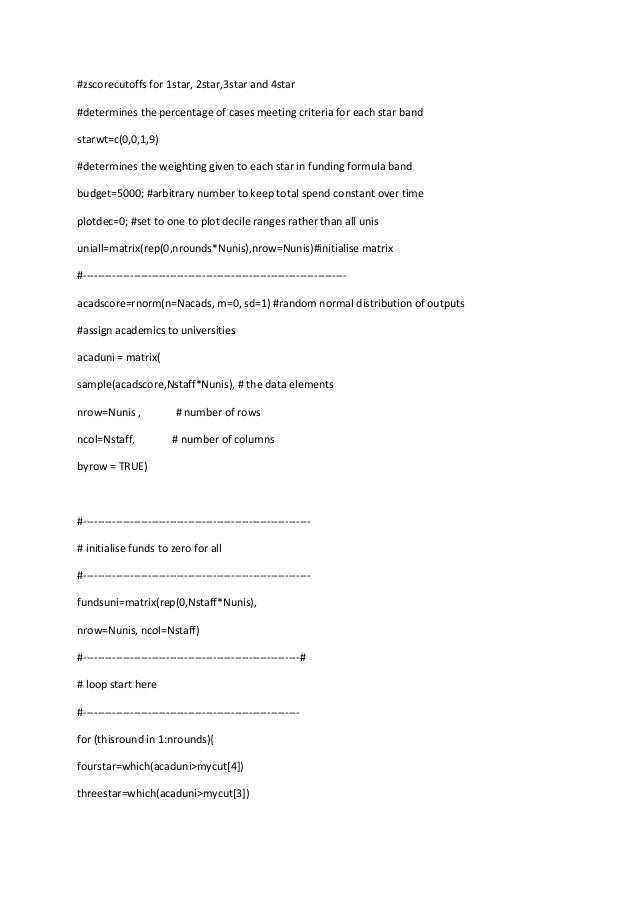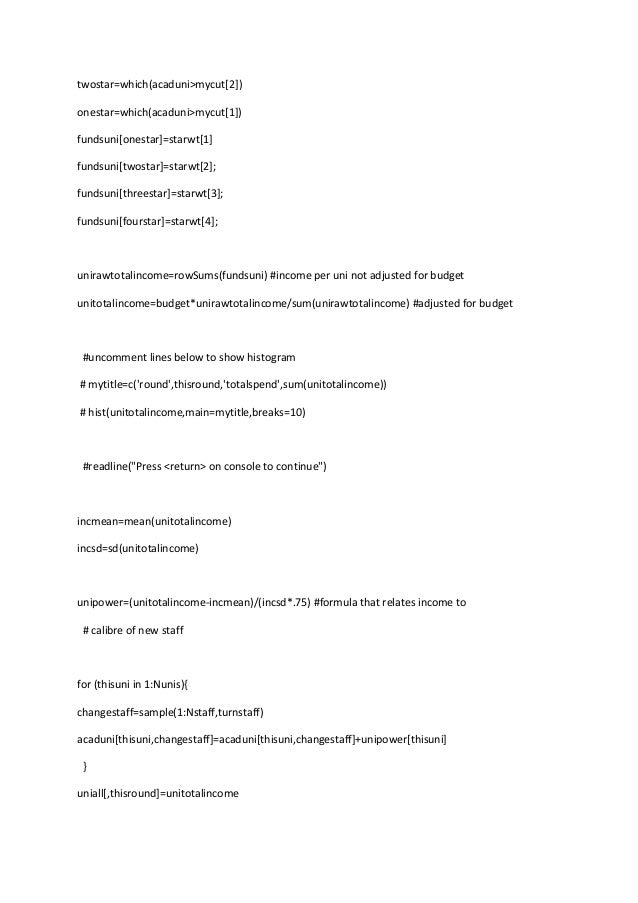Successfully reported this slideshow.Upcoming SlideShare
×

of0

Share

# Refsim (R program)

Simulation of how funding formula for UK universities creates disparities between institutions over time. See blogpost The Matthew Effect and REF2014 on http://deevybee.blogspot.co.uk/.

See all

### Related Audiobooks

#### Free with a 30 day trial from Scribd

See all
• Be the first to like this

### Refsim (R program)

1. 1. #------------------------------------------------------------------------------------# REFsim by D V M Bishop # Simulates impact of REF on university income # 13/10/13 #------------------------------------------------------------------------------------# Plots funding income for each university over each funding cycle # Assumes greater funding allows higher-level academics are appointed # You can vary the following: # N universities (Nunis) # Nstaff per university (Nstaff; currently constant for all unis) # turnover ; proportion of staff changing over each cycle # nrounds ; number of cycles of hiring/REF # cutoff; mycut dimension: zscores corresponding to 1*,2*,3*,4* the higher cut is, the fewer will meet 4* # starwt; dimension specifying weighting given to each star level # budget; arbitrary - currently kept constant at each cycle # plotdec; determines whether plot shows all unis or grouped into deciles # results also depend on unipower, which is computed below # unipower determines how spending power relates to calibre of new academics # it's a weighted z-score of income Nacads=10000; #total pool of academics Nunis=100 Nstaff=50 # N universities #Nstaff per uni turnover=.1; #% staff change each run turnstaff=round(turnover*Nstaff) nrounds=6; mycut=c(-1,0,1,2)
2. 2. #zscorecutoffs for 1star, 2star,3star and 4star #determines the percentage of cases meeting criteria for each star band starwt=c(0,0,1,9) #determines the weighting given to each star in funding formula band budget=5000; #arbitrary number to keep total spend constant over time plotdec=0; #set to one to plot decile ranges rather than all unis uniall=matrix(rep(0,nrounds*Nunis),nrow=Nunis)#initialise matrix #------------------------------------------------------------------------acadscore=rnorm(n=Nacads, m=0, sd=1) #random normal distribution of outputs #assign academics to universities acaduni = matrix( sample(acadscore,Nstaff*Nunis), # the data elements nrow=Nunis , # number of rows ncol=Nstaff, # number of columns byrow = TRUE) #--------------------------------------------------------------# initialise funds to zero for all #--------------------------------------------------------------fundsuni=matrix(rep(0,Nstaff*Nunis), nrow=Nunis, ncol=Nstaff) #------------------------------------------------------------# # loop start here #-----------------------------------------------------------for (thisround in 1:nrounds){ fourstar=which(acaduni>mycut) threestar=which(acaduni>mycut)
3. 3. twostar=which(acaduni>mycut) onestar=which(acaduni>mycut) fundsuni[onestar]=starwt fundsuni[twostar]=starwt; fundsuni[threestar]=starwt; fundsuni[fourstar]=starwt; unirawtotalincome=rowSums(fundsuni) #income per uni not adjusted for budget unitotalincome=budget*unirawtotalincome/sum(unirawtotalincome) #adjusted for budget #uncomment lines below to show histogram # mytitle=c('round',thisround,'totalspend',sum(unitotalincome)) # hist(unitotalincome,main=mytitle,breaks=10) #readline("Press <return> on console to continue") incmean=mean(unitotalincome) incsd=sd(unitotalincome) unipower=(unitotalincome-incmean)/(incsd*.75) #formula that relates income to # calibre of new staff for (thisuni in 1:Nunis){ changestaff=sample(1:Nstaff,turnstaff) acaduni[thisuni,changestaff]=acaduni[thisuni,changestaff]+unipower[thisuni] } uniall[,thisround]=unitotalincome
4. 4. } # for plotting sort the unis by income unisorted=(uniall[order(uniall[,1]),] ) pickuni=seq(1,Nunis,by=10)#pick every 10th uni uniall2=(unisorted[pickuni,]+unisorted[pickuni+1,]+unisorted[pickuni+2,]+unisorted[pickuni+3,]+unis orted[pickuni+4,]+unisorted[pickuni+5,]+unisorted[pickuni+6,]+unisorted[pickuni+7,]+unisorted[pick uni+8,]+unisorted[pickuni+9,])/10 mytitle=t(c('Weights: 1*=',starwt,"; 2*=",starwt,'; 3*=',starwt,'; 4*=',starwt)) mydata=uniall #default plot all unis if (plotdec==1){ mydata=uniall2 #otherwise plot decile bands } matplot(t(mydata),type='l',lwd=2,las=1,xlab='Cycle',ylab='Income',main=paste(mytitle,collapse=''),co l=1,lty=2,ylim=c(0,300))

Total views

1,493

On Slideshare

0

From embeds

0

Number of embeds

1

5

Shares

0